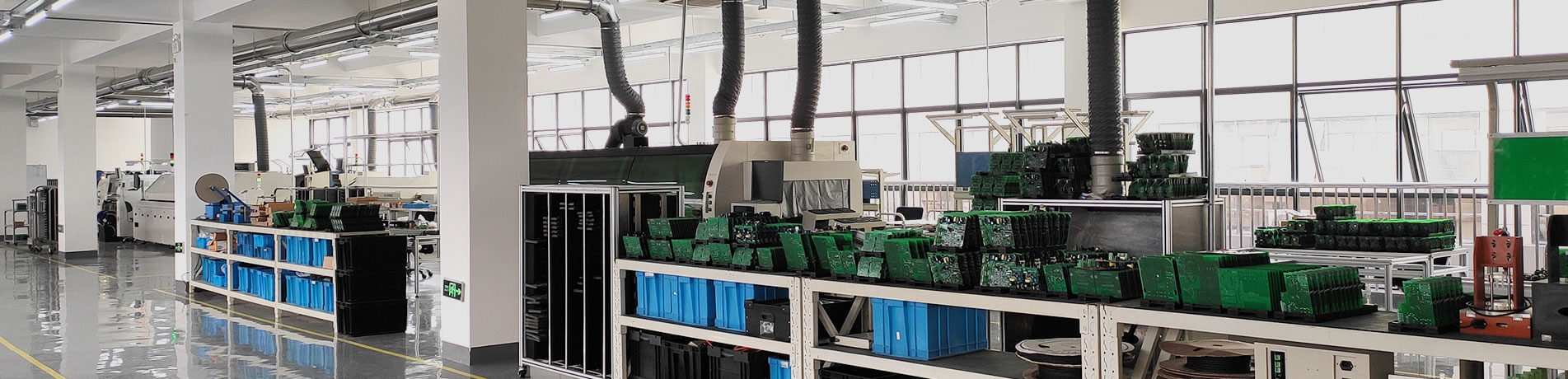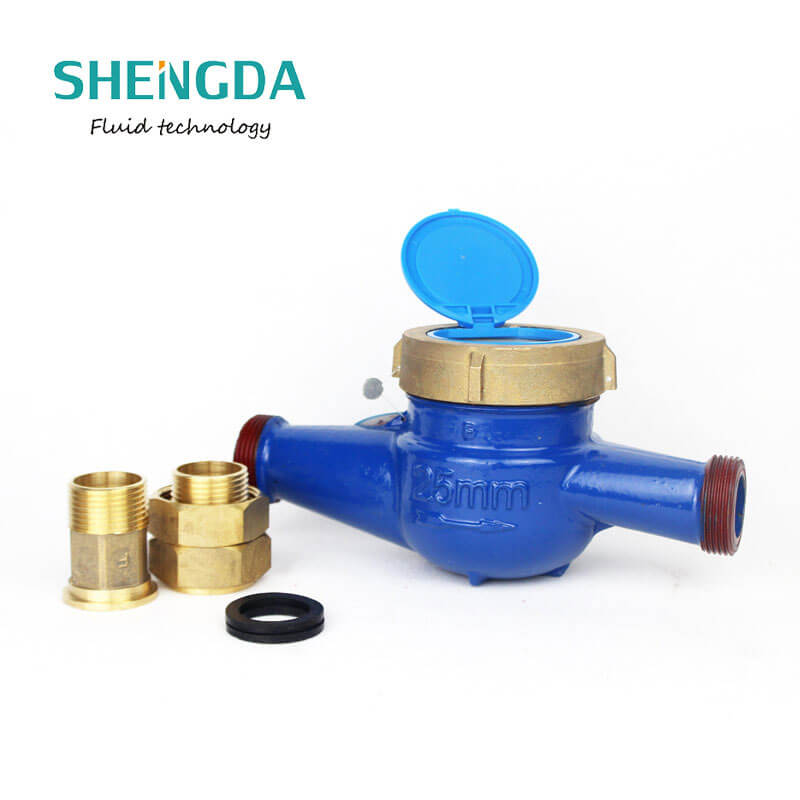## Water meter standards ISO4064 requirements-1

2021-02-25 14:27:44

Water meter standards are inside the ISO 4064 documents. The same documents are named EN, for European Norm, 4064-1 2-6. And they are relevant for whole EMEA. In fact, the ISO documents have been also adapted under different name for the Australia region as well.

And all water meter devices in Europe today are based on these standards where all relevant parameters for water meter device are defined. We will look at the few most important ones. Obviously, in a meter, we are mostly interested about the flow rate, Q, where V is the volume, and t is the time taken for this volume to pass through the meter.

The permanent flow rate, Q3, is the highest flow rate within the rated operating conditions at which the meter is to operate within the maximum permissible errors. Flow rate is expressed in cubic meters per hour.

We have also an overload flow rate, Q4, which is the highest flow rate at which the meter is to operate for a short period of time, within the maximum permissible errors while maintaining its metrological performance when it is subsequently operating within the rated operating conditions, which is Q3.

As mentioned, these definitions are found in the EN ISO 4064-1 from 2014, November. The transition flow rate, Q2, is the flow rate which divides the flow rate range into two zones, the upper flow rate zone and the lower flow rate zone, each characterized by its own maximum permissible errors.

The minimum flow rate, Q1, is the lowest flow rate at which the meter is to operate within the maximum permissible errors. And then, we have several other parameters, like the minimum admissible temperature, the maximum admissible temperature, working temperature, and the working pressure, which is the average water pressure, or gauge.

Water meter flow rates– the flow rate characteristics of a water meter is defined by the values of Q1 through Q4. And a water meter is typically characterized by the numerical value of Q3 in cubic meters per hour and the ratio of Q3 to Q1. This represents the highest flow and the minimum flow rate of the water meter device.

And for Q3, we typically have a list of popular values to choose from. And for the residential types, these are typically the 1.6, 2.5, and 4. The ratio of Q3:Q1 is typically chosen from the list shown here where the factor 80, or 100 to 160, are the most popular ones.

The ratio Q2:Q1 shall be 1.6. That’s the transitional to minimum ratio. And the ratio Q4:Q3 shall be 1.25, which means that the short-term overload is 25%.